type="text/css" />

### SquareRoot2TimesSquareRoot8 General forum

21 replies. Last post: 2021-04-14

SquareRoot2TimesSquareRoot8
•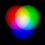Enforce Aspiration of Vaccine at 2020-12-12

My second question that I ask maths teachers, everyone so far has got wrong

What is the square root of 2 times the square root of 8

P.S. extra points available

•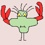BartSimpson4321 at 2020-12-12

√2√8

Approximately 1.4 times approximately 2.8

3.9.

Was that close? I used a calculator and rounded to the nearest tenth.

•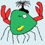William Fraser at 2020-12-12

One interesting question is whether you mean sqrt(2)*sqrt(8) or sqrt(2*sqrt(8)).  The former is 4, while the latter is 2*sqrt(sqrt(2)) or 2*(fourth root of 2).

•William Fraser at 2020-12-12

Drat that formatting.  Just fill in the missing star where the bold starts and another where the bold ends.

•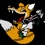metzgerism at 2020-12-12

depends on the context…

•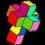ypercube at 2020-12-13

William is correct. The question can be interpreted to ask for either:

- (the square root of 2) times (the square root of 8)

or:

- the square root of (2 times the square root of 8)

Everyday talk can be ambiguous when discussing mathematics.

And William is correct that the first question yields sqrt(16) or 4

while the 2nd yields fourth-root(32) or 2 x fourt-root(2)

(under the most commonly found definition of square root.

Noting that, the most common definition of square root of a real number a is positive (or zero) real solution to the equation x^2 = a.

This equation has no real solutions for negative a.

For a=0, there is the (double) solution of x=0.

And for positive a has two real solutions, one negative and one positive. The latter (positive) is most mathematics books is defined as the square root of a or sqrt(a) or √a.

Now, there are a few (and not common at all) books where the square root of a real (or complex) number a is defined as any solution to the above equation.

This results in a rather confusing situation.

For positive a - and in those books/definition), the square root of a is either (the positive) √a or (the negative) -√a.

And for negative a, we also have two (complex solutions) this time: i sqrt(\|a\|) and -i sqrt(\|a\|).

(where \|a\| denotes the absolute value of a and i is the complex unit, aka one of the two solutions to the equation x^2 = -1)

That makes our problem - and only by this rarely used definition of square root - to have a different/more solutions:

- (the square root of 2) times (the square root of 8)

or:

- the square root of (2 times the square root of 8)

And now we get that the the first question yield  (sqrt(16) = 4) OR (-sqrt(16) = -4). So we have two solutions.

while the 2nd yields the square root of (4 sqrt(2) ) OR the square root of ( -4 sqrt(2) ) and this gives us four solutions:

sqrt(4 sqrt(2)) = fourth-root(32) =  2 fourth-root(2)

OR

-sqrt(4sqrt(2)) = - fourth-root(32) =  -2 fourth-root(2)

OR

i sqrt(4 sqrt(2)) = i fourth-root(32) =  2i fourth-root(2)

OR

-i sqrt(4sqrt(2)) = -i fourth-root(32) =  -2i fourth-root(2)

In conclusion, if a math teacher gave you the first (William's) answer, I find it ok. Not perfect but that's all I'd expect from someone teaching in primary school or the first grades of secondary school.

The 2nd answer, I'd expect to be given by someone who has a university degree in maths or at least has been taught or has been exposed to complex analysis. And I think that most countries do not expose pupils in secondary schools to complex analysis or even introduce the complex numbers.

•Enforce Aspiration of Vaccine at 2020-12-13

Answer can be displayed using a handful of matchsticks, tooth picks, pens, pencils, lollies sticks, old remote controls, fence posts or trained stick insects

My future version will be “what IS the square root of minus 2 times the square root of minus 8” (with very little emphasis of IS)

•Enforce Aspiration of Vaccine at 2020-12-13

Even better “what IS minus the square root of minus 2 times minus the square root of minus 8”

•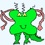ypaul21 ★ at 2020-12-13

I think that the only grammatically correct interpretation of that statement is sqrt(2*sqrt(8)), if you’ll hear me out.

I’ve been learning APL recently, and I’ve started to get used to it’s very order logical order of operation that starts with the right-most operator.

Basically, the argument is that in normal English, you’d most interpret it naturally that way. Consider the statement “Take the top-half of the bottom half”. The most natural way to interpret this is to “take the bottom half first, then take the top-half of this bottom half”, rather than to “take the top half first, then take the bottom half of the top half”.

Similarly for binary operations, when you say “2 times 3 plus 4”, you actually mean 2*(3+4). If you intended to say (2*3)+4, you’d have to say something along the lines of “2 times 3, the result of which plus 4”.

Interpreting the former as (2*3)+4, I would argue, is just a result of reading out the names of the constants/variables and the operators as they appear, and so it makes sense in some way, but is actually ungrammatical. That the reason why people tend to end up saying it with an awkward pause after saying the 3, precisely because they subconsciously know that it’s incorrect (and you will not find any logical place to put an equivalent pause if you meant the other statement).

Of course, there are other ways to read it that may be much clearer, like “the product of 2 and the sum of 3 and 4” vs “the sum of the product of 2 and 3 and 4”, etc but that’s beside the point.

This follows with any number of arguments and operations, whether they involve unary operators like the square root, or binary operators like multiplication. When you read a sequence from left to right, you’d perform the operations starting from the right end.

•Enforce Aspiration of Vaccine at 2020-12-13

Usual order of operation , powers first then multiply

KISS

•ypaul21 ★ at 2020-12-13

The order of operation is arbitrarily defined, and it’s done in a way that’s most convenient for our notation. I’m talking about it purely in terms of how it’s naturally interpreted grammatically in the English language.

If you were to say the “fourth power of three minus one”, it should be interpreted as (3-1)⁴.

•ypaul21 ★ at 2020-12-13

Oh yeah, something else that’s worth pointing out: It’s wrong to say the square root of x because the use of the definite article together with the singular ‘root’ implies that you’re talking about a specific root, but it’s not clear which one you are referring to. Some correct statements would be

• The product of the principal square root of x and the principle square root of y
• The product of x square root of a and a square root of y
• The products of the square roots of x and the square roots of y

“The product of the square root of x and the square root of y” is nonsensical unless you’re implicitly defining the ‘square root; to mean the principal square root.

•ypaul21 ★ at 2020-12-13

Sorry, I initially used ‘a’ and ‘b’ and only changed them to ‘x’ and ‘y’ at the last minute, and I accidentally swapped the wrong ‘a’ with ‘x’ in my second bullet point.

•Enforce Aspiration of Vaccine at 2020-12-13

The only trick in grammar is to use IS to misled, it should be ARE

•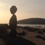Carroll ★ at 2020-12-13

This discussion reminds me about the question: “which is the real (sic) solution to z^2= -1, is it 'i' or '-i', is it the +90° imaginary unit or the -90° imaginary unit ?”

Can you design a real coefficient equation to tell them apart (i will be a solution but not -i) ?

•William Fraser at 2020-12-14

IIRC, all non-real roots to polynomials with real coefficients show up in conjugate pairs.  In other words, no you can't.

•Carroll ★ at 2020-12-14

Yes for polynomials you can't, what about other equations?

My point is that i was chosen by convention, -i could have been chosen instead, or you can look at the complex plane from beneath instead as from above… if those terms have any signifiance.

•ypaul21 ★ at 2020-12-14

If other equations are allowed, then you could probably just specify it by using some sort of reference, like for example, minimisation of its distance with respect to another chosen complex number.

•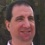Dvd Avins at 2020-12-30

Among native English speakers from the same part of the world, inflection should make it obvious which question is being asked. (And universities would do well to only assign classroom duties to instructors who speak closely enough to the way their students do so that this will not become a problem.)

•ypaul21 ★ at 2020-12-31

The point that I was trying to make is that it’s artificial. You’d raise the pitch or add a pause right after a term where you’re breaking this law in order to add an imaginary bracket in the expression to make it clear where the demarcation is. I’m not saying that it’s not done or if it’s necessarily unnatural, but it’s just more natural to parse it in the manner that I had described.

In most practical instances though, this won’t be a problem at all as you’d be able to tell which one you’re implying based on context. You’d rarely need to talk about a random expression outside of elementary maths problems. And even when you do, you can usually find some way to put it into writing such that it wouldn’t cause any potential confusion.

•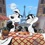CleverHunk steven cullum at 2021-04-14

“To find the square root of a third,

Is clearly extremely absurd.

Every math student knows,

The way that it goes:

You' ll always end up with a surd.”